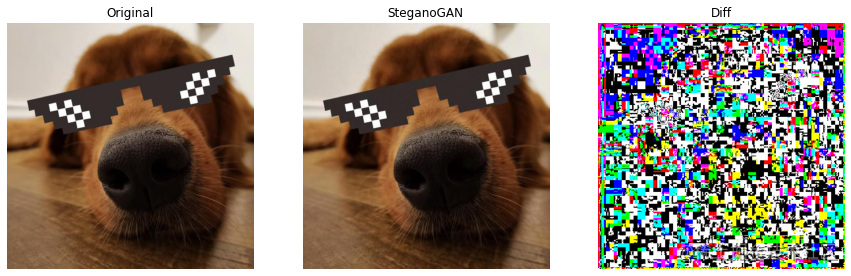# 图像隐写技术（Image Steganography）

### 元数据修改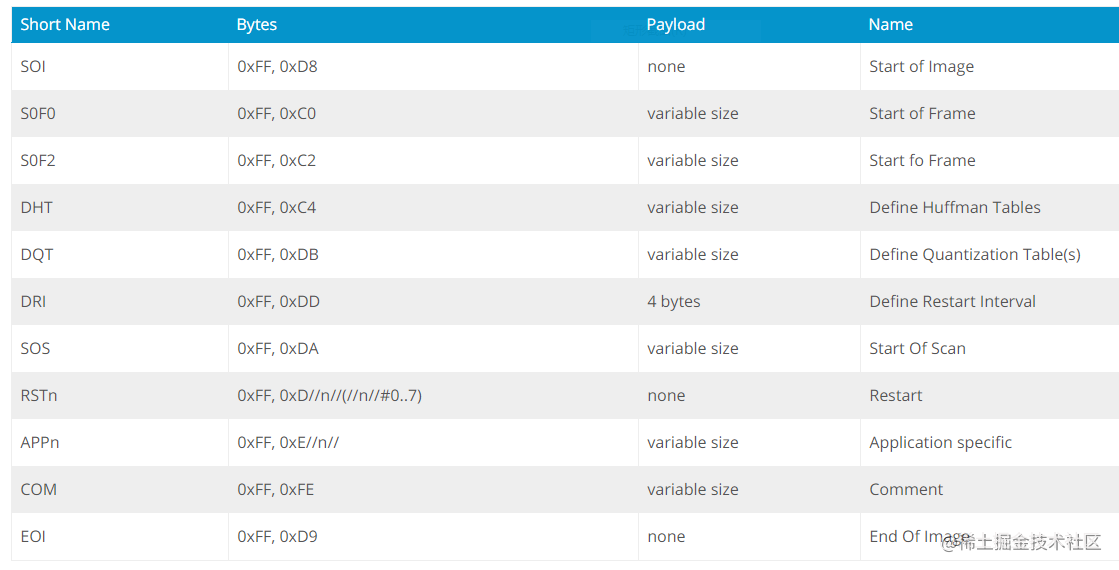``````with open("input.jpg", "rb") as f:
f_bytes = f.read()
print( f_bytes[:2] )
print( f_bytes[-2:] )

# OUTPUT
"""
b'\xff\xd8'
b'\xff\xd9'
"""

``````txt = '你好 PyHub!'

# 编码
with open("out_append.jpg", "wb") as f:
with open("input.jpg", "rb") as ff:
f.write(f_bytes[sos:])
f.write('你好 PyHub!'.encode())

# 解码
with open("out_append.jpg", "rb") as f:
content = f.read()
eoi = content.find(b'\xff\xd9')
print(content[eoi+2:].decode())

# OUTPUT
"""
你好 PyHub!
"""

### 比特操作

``````from PIL import Image
import numpy as np
from matplotlib import pyplot as plt

logo = Image.open("logo.jpg").convert("L")
logo_bin = np.where( np.array(logo) > 128, 1, 0).astype(np.uint8)``````base = Image.open("input.jpg")
# 最低位变为 0
base_lsb = np.bitwise_and(base, 0xFE)
# 将 logo 拼接到最低位(其中一个通道，也可以保留3个通道)
logo_h, logo_w = logo_bin.shape
base_lsb[:logo_h, :logo_w, 0] += logo_bin

# 解码
base_decode = np.bitwise_and(base_lsb[:,:,0], 1)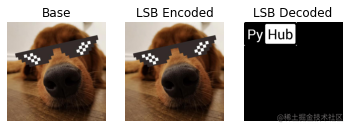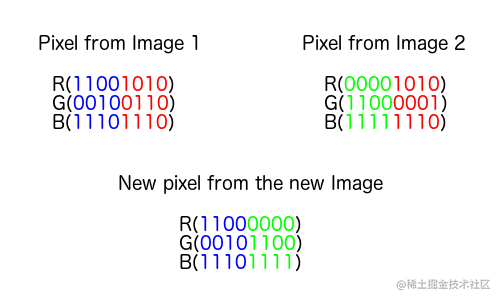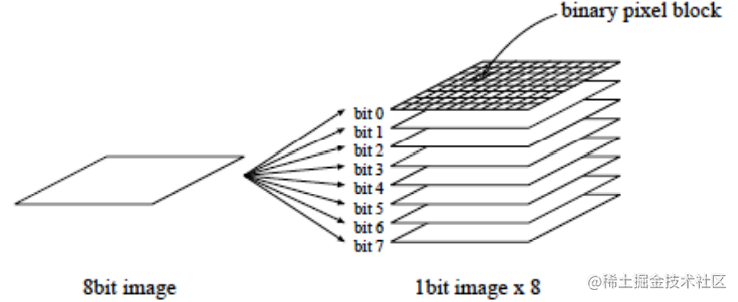### 频域水印

``````# 原图，1-1
base = Image.open("input.jpg").convert("L")

base_fft = np.fft.fft2(np.array(base))
base_ffs = np.fft.fftshift(base_fft)

# 绘制水印
fnt = ImageFont.truetype("Cyberway Riders.otf", 40)
wm = Image.new("L", base.size, (0))
ImageDraw.Draw(wm).text((0,0), "PyHub", font=fnt, fill=(255))
wm_arr = np.array(wm)
# 反转生成中心对称，图1-2
fft_wm_arr = (np.flip(wm_arr) + wm_arr).astype(np.uint8)

# 叠加水印，图1-3
base_ffs.real[fft_wm_arr == 255] = 255

# 傅里叶逆变换，图2-1
base_reversed = np.real( np.fft.ifft2( np.fft.ifftshift(base_ffs) ) )
# 对逆变换（编码）后的图进行解码，图2-2
base_decode = np.clip(np.fft.fftshift( np.fft.fft2(base_reversed)).real, 0, 255)

# 编码后的图与原图的差异，图2-2
base_reversed - np.array(base)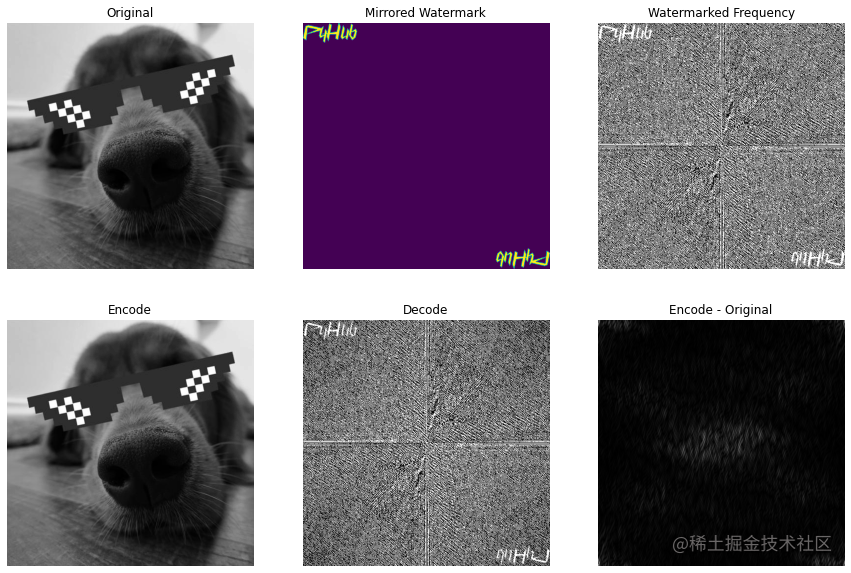### 深度学习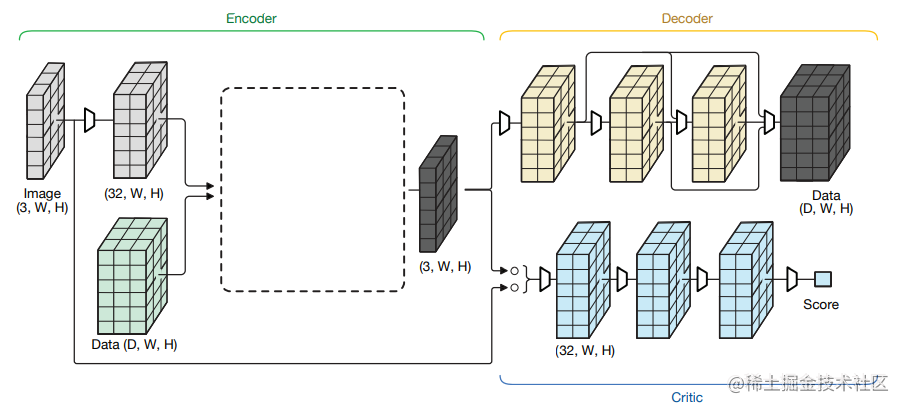``````steganogan encode input.jpg "Hi PyHub" -o out_gan.jpg